Latest SSC jobs   »   Compound Interest Formulas

# Compound Interest Formulas, Tricks And Questions

## Compound Interest Formulas, Tricks, And Questions

Compound Interest Formula: The questions based on the compound interest calculate the interest on interest, based on the initial principal. Compound Interest is an interesting topic that helps you to calculate interest in your daily life. Various competitive examinations such as SSC, Banking, CAT, MAT, etc ask the Compound interest questions in its aptitude section compulsorily.

## Compound Interest Tricks

You can learn shortcut methods, Compound interest formulas, and compound interest tricks to solve the questions on this topic. To score good marks in any exam, it is necessary to have knowledge of the important topics and clear the basic concepts. Know about the Compound Interest Formulas, Compound Interest Tricks, and questions based on Compound interest provided below.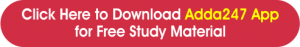### Compound Interest: Formulas & Tricks

How do we calculate the compound interest on a given amount? It can be challenging to get the right answer without knowing the right formula and tricks. To calculate the compound interest, you need the Principal amount, rate of interest, and time period. Check out the formula for compound interest with the example to know in detail.

1. (a) The amount A due after t years, when a principal P is given on compound interest at the rate R% per annum is given by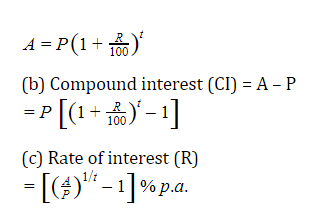Note: Simple interest and compound interest for 1 year at a given rate of interest per annum are always equal.

1. If the interest is compounded half-yearly, then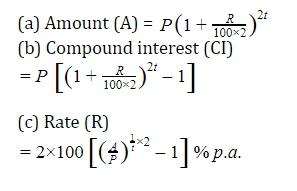1. If the interest is compounded quarterly, then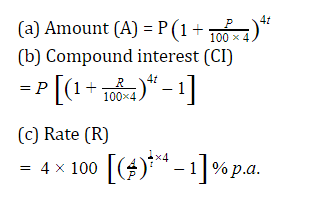In general, if the interest is compound n times a year, then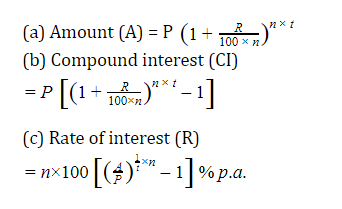1. When the rates of interest are different for different years, say R₁, R₂, R₃ percent for the first, second and third year, respectively, then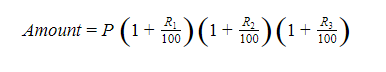1. When the time is given in the form of a fraction, say 2 3/4 years, then,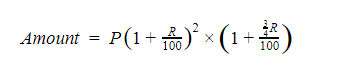1. (a) The difference between the compound interest and the simple interest on a certain sum of money for 2 years at R% per annum is given by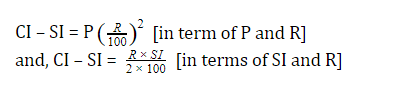(b) The difference between the compound interest and the simple interest on a certain sum of money for 2 years at R% per annum is given by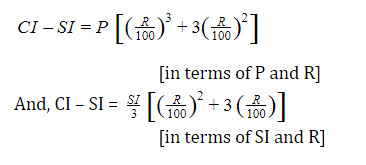1. If a certain sum becomes n times in t years at compound interest, then the same sum becomes n^m times in mt years.
1. If a certain sum becomes n times in t years, then the rate of compound interest is given by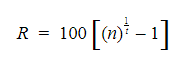1. If a certain sum of money at compound interest amounts to Rs. x in A years and to Rs. y in B years, then the rate of interest per annum is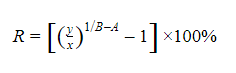1. If a loan of Rs. P at R% compound interest per annum is to be repaid in n equal yearly installments, then the value of each installment is given by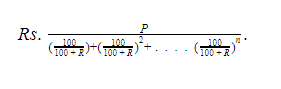### Compound Interest Questions

As we have provided the important formulas related to Compound Interest, here are some questions based on Compound interest with answers that you can practice for a better understanding.

Q1. Rs. 2500 was borrowed for 3 years. What will be the compound interest if the rate of interest for the first year is 3% per annum, the second year is 4% per annum and the third year is 5% per annum respectively?
(a) 311.90
(b)440
(c) 450
(d) 410.80Q2. A sum of money becomes 16 times itself in 2 years if compounded half-yearly. How much time it will take to become 27 times if compounded yearly.
(a) 3 years
(b) 4 years
(c) 5years
(d) 6 yearsQ3. The difference between S.I and C.I on an amount of Rs. 30000 for 2 years is Rs. 147. What is the rate of Interest?
(a) 8 %
(b) 10 %
(c) 9 %
(d) 7 %Q4. If the ratio of the difference between S.I and C.I. for two years and 3 years is 4:13. Find the rate of interest.
(a) 20 %
(b) 25 %
(c) 30 %
(d) 40 %Q5. Rs. 39030 is divided between ‘a’ and ‘b’ in such a way that the amount given to ‘a’ on C.I. in 7 years is equal to the amount given to ‘b’ on C.I. in 9 years. Find the part of ‘a’. If the rate of interest is 4%.
(a) 20200
(b) 20900
(c) 20280
(d) 20100Q6. What will be the difference between simple and compound interest on a sum of Rs. 4500 put for 2 years at 5% per annum?
(a) 12.45
(b) 12.95
(c) 11.25
(d)10.25Q7. What will be the amount if a sum of Rs. 5000 is placed at compound interest for 3 years while the rate of interest for the first, second, and third years is 2%, 3%, and 4% respectively?
(a) 5473.12
(b) 5463.12
(c) 5163.12
(d) 5353.12Q8. The compound interest on Rs. 10,000 in 2 years at 4% per annum the interest being compounded half-yearly, is :
(a) 824.3216 Rs
(b) 804.3216 Rs
(c) 814.3216 Rs
(d) 834.3216 RsQ9. The compound interest on a certain sum of money invested for 2 years at 5% per annum is Rs. 328. The simple interest on the sum, at the same rate and for the same period will be?
(a) 340
(b)320
(c)330
(d)390Q10. A sum of Rs. 2000 amounts to Rs. 4000 in two years at compound interest. In how many years does the same amount become Rs. 8000.
(a) 2
(b) 4
(c) 6
(d) 8## Compound Interest FAQ

Q. What is Compound Interest?

Ans: Compound interest is the interest on a loan or deposit that accrues on both the initial principal and the accumulated interest from previous periods.

Q.How do we calculate compound interest?

Ans: We can calculate CI by using the formula for compound interest is A = P(1 + r/n)^nt.

Q.What is compound Interest with examples?

Ans. The compound interest examples are explained here.

###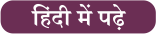#### Congratulations!

General Awareness & Science Capsule PDF